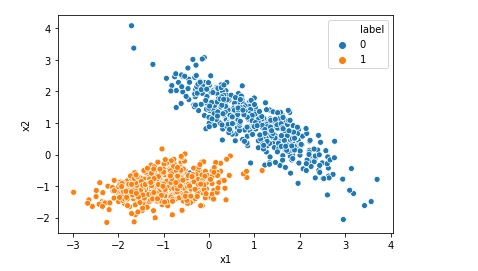# What is R Squared for Linear Regression

For Linear Regression, R-squared is a statistical term which indicates how close the data are to the fitted regression line. R-Squared is also known as coefficient of determination. R-squared = Explained variation in data / Total variation in data R-squared = 1 - (RSS/TSS) RSS = Sum of squares of difference between predicted value and … Continue reading What is R Squared for Linear Regression# What is Precision, Recall and F1 Score

Precision, recall and F1 Score are parameters to measure the performance of a classification model. For example if we want to implement Logistic Regression model on an imbalanced dataset we would like to calculate precision, recall and F1 score as accuracy may not be a good measure of model performance in this case. Precision: Precision … Continue reading What is Precision, Recall and F1 Score# What is Confusion Matrix in Machine Learning

Not only human beings but also the machine learning models may get confused !! After all Artificial Intelligence mimics a human brain, isn’t it?(pun intended). Imagine yourself as a machine learning engineer and suppose you trained a machine learning classification model successfully today. After the model is trained you checked the accuracy which is 93.0%. Wow … Continue reading What is Confusion Matrix in Machine Learning# Implementing Logistic Regression in 10 lines in Python

Logistic Regression is one of the most popular Machine Learning algorithm used for the classification problems. It should be noted that though there is a regression word in the name of the algorithm Logistic Regression, it is used for classification problems. A use case of Logistic regression could be, based on the symptoms for a disease that a patient has Logistic … Continue reading Implementing Logistic Regression in 10 lines in Python